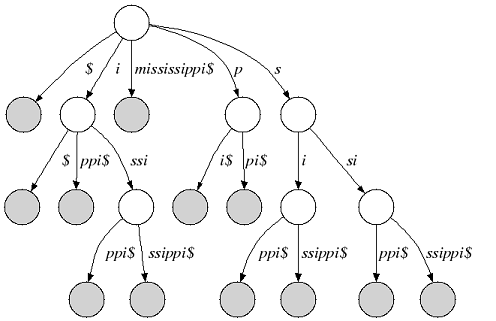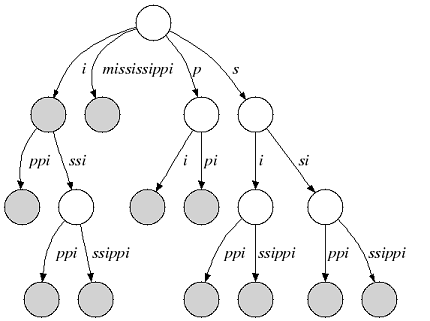# Suffix Tree¶

We consider an alphabet Σ and a sentinel character $that is smaller than every character of Σ. A suffix tree of a given non-empty string s over Σ is a directed tree whose edges are labeled with non-empty substrings of s$ with the following properties:

1. Each outgoing edge begins with a different letter and the outdegree of an internal node is greater than 1.
2. Each suffix of s$is the concatenation of edges from the root to a leaf node. 3. Each path from the root to a leaf node is a suffix of s$.

The following figure shows the suffix tree of the string s=”mississippi” (suffix nodes are shaded):Figure 1: Suffix tree of “mississippi”

Many suffix tree construction algorithms expect $to be part of the string alphabet which is undesirable for small bit-compressible alphabets (e.g. DNA). In SeqAn there is no need to introduce a$. We relax suffix tree criterion 2. and consider the relaxed suffix tree that arises from the suffix tree of s by removing the \$ character and all empty edges. In the following, we only consider relaxed suffix trees and simply call them suffix trees. In that tree a suffix can end in an inner node as you can see in the next figure (suffix “i”):Figure 2: Relaxed suffix tree of “mississippi”